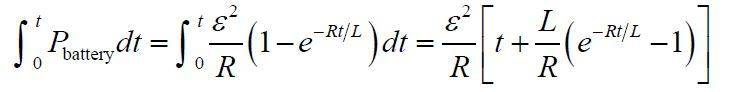# Power delivered by battery in simple RL circuit

Taulant Sholla

## Homework Statement

For a simple series LR circuit, find the energy delivered to the circuit by the battery.

## Homework Equations

I = (V/R)*(1-e-Rt/L)
P=IV
E=∫Pdt

## The Attempt at a Solution

Isn't ∫ Pdt = ∫ IVdt = ∫ (V2/R)*(1-e-Rt/L)dt = (V2/R)(t + (L/R)e-Rt/L) ?

The book provides the answer, via a sample problem, of:What am I missing?